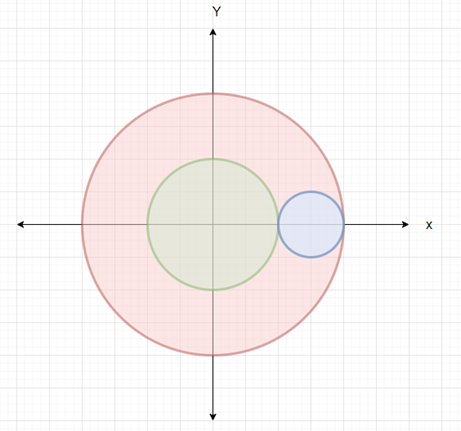# Check whether given circle resides in boundary maintained by two other circles in Python

Suppose we have two radius values r1 and r2 of two concentric circles. We have another input coordinate coord and a radius value r. We have to check whether the circle whose center is placed at coord and it fits inside the boundary of two given concentric circles.

So, if the input is like r1 = 4 r2 = 2 coord = (3, 0) r = 1, then the output will be True.To solve this, we will follow these steps −

• val := square root of(x^2 + y^2)
• if val + r <= r1 and val - r >= r1 - r2, then
• return True
• return False

Let us see the following implementation to get better understanding −

## Example Code

Live Demo

from math import pow, sqrt

def solve(r1, r2, coord, r) :
val = sqrt(pow(coord, 2) + pow(coord, 2))

if val + r <= r1 and val - r >= r1 - r2 :
return True
return False

r1 = 4
r2 = 2
coord = (3, 0)
r = 1
print(solve(r1, r2, coord, r))

## Input

4,2,(3, 0),1


## Output

True

Updated on: 16-Jan-2021

78 Views